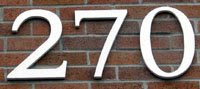## Friday, April 3, 2009

### 270

270 = 2 x 33 x 5.

270 is a number n such that n2 + 1 is prime: 2702 + 1 = 72901, a prime.

270 is the smallest number whose sum of divisors (720) equals 10 times its reverse.

10! has
270 divisors.

270 is a number n such that 6n - 1 and 6n + 1 are twin primes: 6*270 - 1 = 1619 and 6*270 + 1 = 1621.270 is one the standard numerical values for resistors.

Source:
Number Gossip